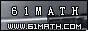Today is . WELCOME: huohai3983/44663  Home | Reg Login | All user | setHomePage | BookmarkHome | Math Test Online | Addition | Subtraction | Multiplication | Division | Time | Worksheet(JPG/PDF) | CAI | Flash Games |Recommendation articleArticle searchHome--maths--Third Grade--Adding Numbers Arranged Vertically

How to add two two-digit numbers (for example 29 + 33).

• If the numbers are arranged vertically, they are in a format ready to be added.
```
29
33

```
• Add the ones' place digits (9 + 3 = 12). This sum is a two-digit number, so place a one above the tens' place column and place the two below the line in the ones' place column.
```
1
29
33
2
```
• Add the tens' place digits (1 + 2 + 3 = 6) and place the answer below the line in the tens' place column.
```
29
33
62```

#### Find the Sum of the two Numbers.

 -------

2006-11-04 20:39:54
Viewed 2470 times CloseGreatmathsites